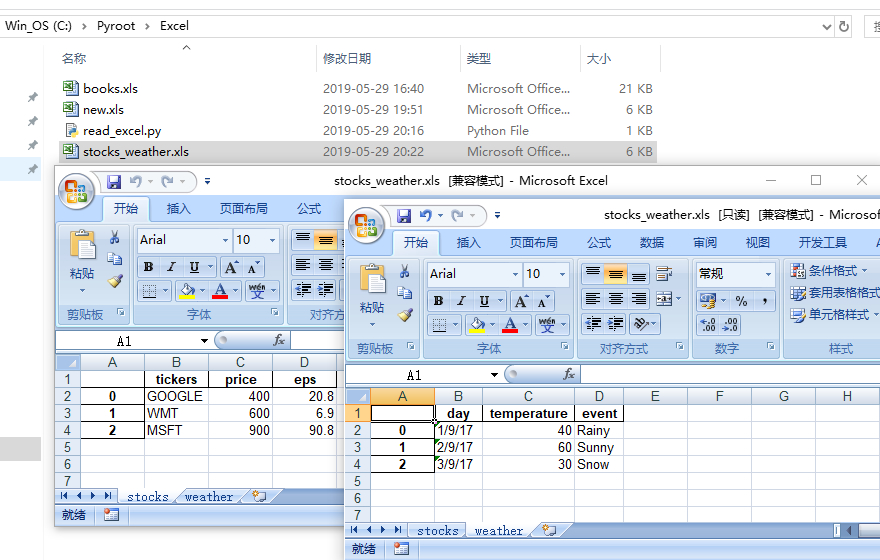• Excel  是微软出品的和款办公软件
• 它能够创建和编辑以”xls”和”xlsx”为后缀的电子表格文件
• Excel文件可以在Windows、macOS、Android和iOS系统中使用
• 自1993年发布5.0 版本以来，Excel 已经成为电子表格事实上的标准

Excel 的用途

• 绘图
• 数据透视表
• 单元格计算

Python读取Excel文件教程

1. 创建一个新项目

2. 创建一个Excel文件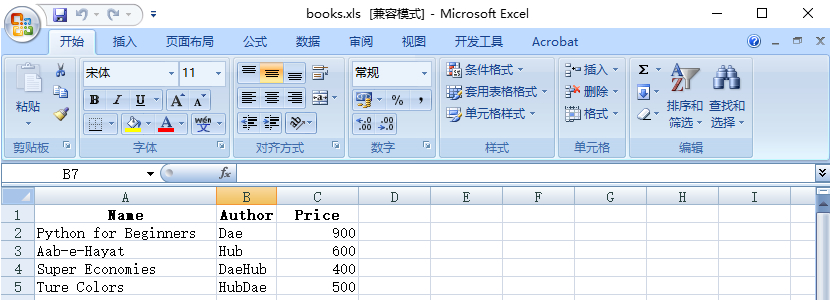3.  安装Python 库

• Pandas 是基本NumPy 的软件库，可以提供易于使用的Python 数据结构和数据分析工具
• Pandas 不仅强大而且非常灵活，经常被用于数据分析

`pip install pandas`

`pip install xlrd`

4. 读取Excel文件

```import pandas as pd

file = "books.xls"

print(data)```

• 首先引入pandas模块
• 最后在终端将data变量的内容打印出来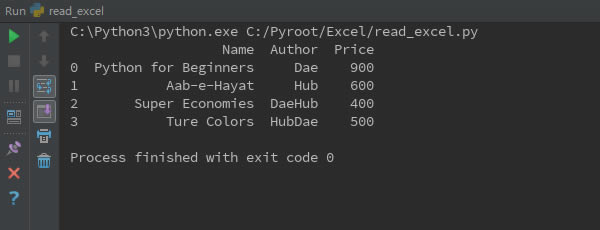5.  修改读取内容

```import pandas as pd

file = "books.xls"
def convert_author_cell(cell):
if cell == "HubDae":
return 'Daehub'
return cell

print(data)```

• 首先定义一下转换单元格内容函数”convert_author_cell(cell)”，该函数以读取的单元格为参数，返回值同样为单元格
• 通过”convert_author_cell(cell)”函数将读取的单元格内容进行转换
• 在读取Excel文件时，会对”Author”字段调用”convert_author_cell(cell)”函数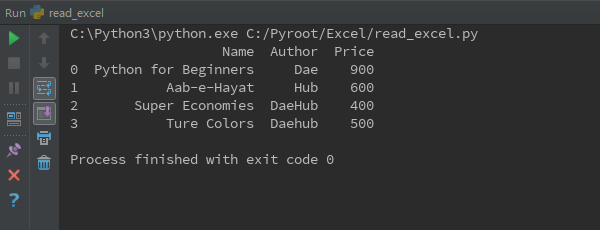6.  Python写入Excel文件

`pip install xlwt`
• xlwt是用于创建兼容Excel 2003的软件包
• xlwt本身是由Python标准包编写完成，不需要其他任何依赖包

```import pandas as pd

file = "books.xls"

#writing excel file
data.to_excel("new.xls", sheet_name="Student")```

• 为了写入Excel文件，需要调用”to_excel”方法
• 该方法接受两个参数，其中一个为写入的文件名，示例为”new.xls”，另一个为工作薄名也就是Excel中的sheet名，示例为”Student”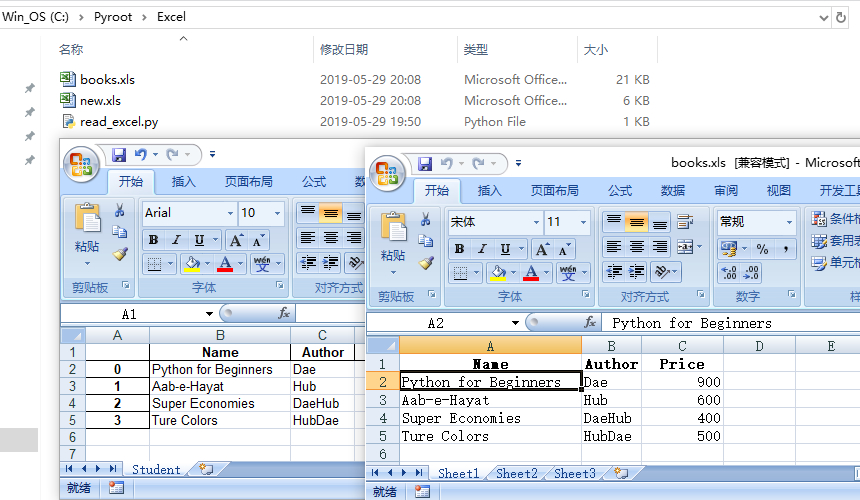7.  将不同数据段数据写入同一个Excel文件中的不同工作薄

```import pandas as pd

#creating dataframes
df_stock = pd.DataFrame({
'price':[400,600,900],
'eps':[20.80,6.90,90.8]
})

df_weather = pd.DataFrame({
'day':['1/9/17','2/9/17','3/9/17'],
'temperature':[40,60,30],
'event':['Rainy','Sunny','Snow']
})

with pd.ExcelWriter('stocks_weather.xls') as writer:
df_stock.to_excel(writer,sheet_name="stocks")
df_weather.to_excel(writer,"weather")```

• 首先调用DataFrame方法创建两个数据对象
• 然后调用ExcelWriter方法创建一个写Excel文件对象
• 最后调用数据对象的to_excel方法将数据写入到Excel文件中，并且将对应的工作薄命名为”stocks”和”weather”